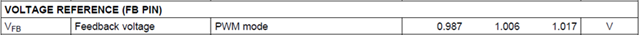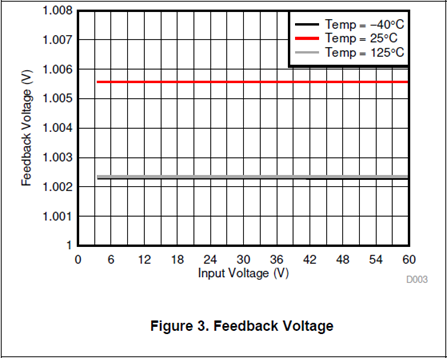If you have a related question, please click the "Ask a related question" button in the top right corner. The newly created question will be automatically linked to this question.

# LM76002-Q1: Specification of 'Voltage Reference (FP)'-Pin

Part Number: LM76002-Q1

Hello Team, not sure if this can be compared but in our datasheet we have:VFB (min) = 0.987 V

VFB (typ) = 1.006 V

VFB (max) = 1.017 V

Chapter 6.9 there is a graphic which shows different valuesVFB (min) = 1.0023 V

VFB (typ) = 1.0 V?

VFB (max) = 1.0056 V

Is there any reason for this deviation and which values can be used for a 'worst-case' calculation?

Many Thanks

Josef

• Hi Josef,

I do not know the exact reason of this 1.5% deviation between values, as this can be a combination of test board, equipment calibration, etc. But I would recommend to use the electrical characteristics values for worst-case. The values found in the electrical characteristics of datasheet are provided for reference and represent the most likely parametric norm.

Regards,
Alejandro